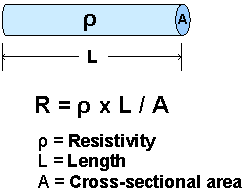# Conductors and insulators

DC-Circuits > Conductors and insulators

A Conductor is a material that can conduct Electric Current easily, because its Resistance to flow of Current is low. When a potential difference is applied across a conductor, Current can flows through the conductor. This is according to Ohm's Law: Current (I) = Voltage (V) / Resistance (R). Many Conductors are metallic, copper and iron for example. And there are some non-metallic Conductors too, carbon and argon for example.

Resistivity is a unit to measure the oppostions of the materials to the flow of Current. Of all the conductive materials, Silver and Copper are the two most conductive because they have the two lowest Resistivities.

An Insulator is highly resistant to flow of Current, because its Resistivity is extremely high. For example, plastic, wood and glass are Insulators.

Resistivities of some Materials are shown on Table 1.

MaterialResistivity ρ (Ω m)
Silver1.6 × 10-8
Copper1.7 × 10-8
Gold2.4 × 10-8
Aluminium2.7 × 10-8
Brass6.0 × 10-8
Iron1.0 × 10-7
Constantan4.9 × 10-5
Carbon3.5 × 10-5
Wood106 ~ 107
Acrylic> 1013
Plastic 1.0 × 1014

Table 1: Resistivities of Materials

The Resistance of a material of regular cross section can be calculated from the following equation:

R = ρ x L / A

where ρ is the Resistivity of the material, L is the Length, and A is the Cross-sectional Area, Figure 1. The shorter the Length of a material, the smaller the Resistance and the larger the Current flowing through it when potential difference is applied across it. If the Resistivity of the material is extremely small, the change of its Resistance is not obvious when its Length is changed.Figure 1: Relationship between Resistance, Resistivity, Length and Cross-sectional area

DC-Circuits > Conductors and insulators